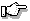This version of this document is no longer maintained. For the latest documentation, see http://www.qnx.com/developers/docs.

# exp(), expf()

Compute the exponential function of a number

## Synopsis:

```#include <math.h>

double exp( double x );

float expf( float x );```

## Arguments:

x
The number for which you want to calculate the exponential.

## Library:

libm

Use the -l m option to qcc to link against this library.

## Description:

The exp() function computes the exponential function of x (ex).

A range error occurs if the magnitude of x is too large.

## Returns:

The exponential value of x.If an error occurs, these functions return 0, but this is also a valid mathematical result. If you want to check for errors, set errno to 0, call the function, and then check errno again. These functions don't change errno if no errors occurred.

## Examples:

```#include <stdio.h>
#include <math.h>
#include <stdlib.h>

int main( void )
{
printf( "%f\n", exp(.5) );

return EXIT_SUCCESS;
}```

produces the output:

`1.648721`

## Classification:

Safety:
Cancellation point No
Interrupt handler No
Signal handler No